Note: The other languages of the website are Google-translated. Back to English

## Excel formula: Count cells greater than or less than

To count the cells greater than or less than a particular number, you can use the COUNTIF function to handle it.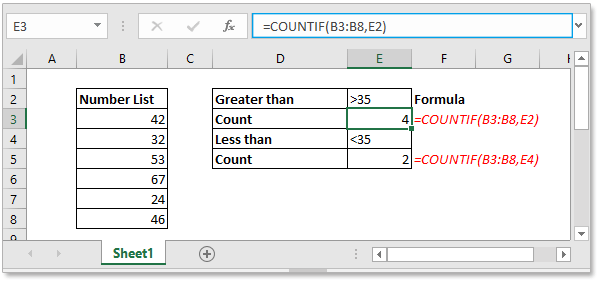### Count cells greater than a particular value

Generic formula:

 COUNTIF(range,criteria)

Example:

Count the number of cells that are greater than 35 from the Number List. Using this formula

 =COUNTIF(B3:B8,E2)

Or

 =COUNTIF(B3:B8,">35")

In above formula, B3:B8 is the range you will count cells from, E2 and “>35” are the criteria you want to count cells based on.

The press Enter key to get the counted result.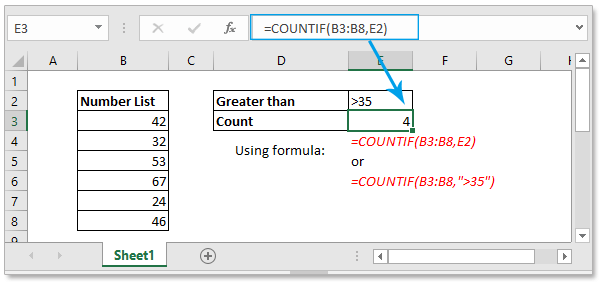Tip:

If you want to count cells greater than or equal to a particular value, you can use this generic formula:

 COUNTIF(range,”>=number”)

Take example: Count cells that greater than or equal to 32, using the formula:

 =COUNTIF(B12:B17,">=32")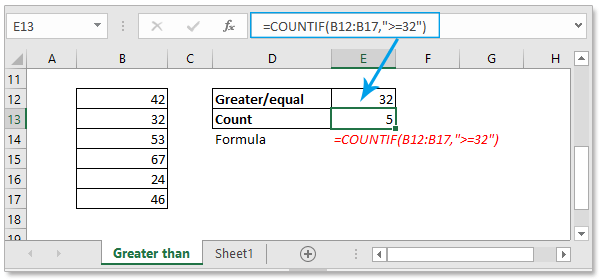### Count cells less than a particular value

Generic formula:

 COUNTIF(range,criteria)

Example

Count the number of cells that are less than 50 from the Number List.

 =COUNTIF(B3:B8,E2)

or

 =COUNTIF(B3:B8,">50")

In above formula, B3:B8 is the range you will count cells from, E2 and “>50” are the criteria you want to count cells based on.

The press Enter key to get the counted result.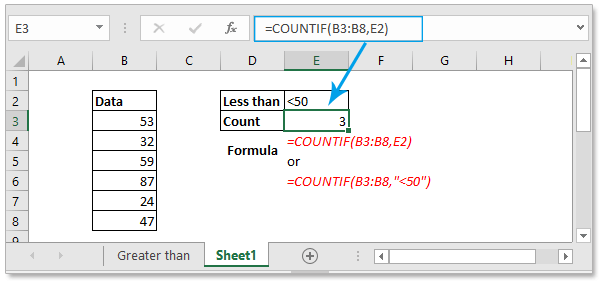Tip:

If you want to count cells less than or equal to a particular value, you can use this generic formula:

 COUNTIF(range,”>=number”)

Take example: Count cells that less than or equal to 59, using the formula

 =COUNTIF(B12:B17,"<=59")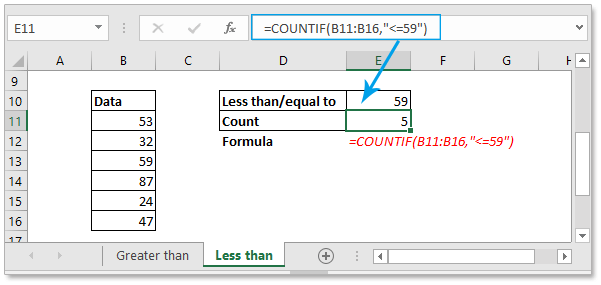### Sample File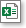Click to download sample file

#### Relative Formulas

• Count cells equal to
With the COUNTIF function, you can count cells that equal to or not contain a specified value.
• Count cells that equal to x or y
In some times, you may want to count the number of cells that meet one of two criteria, in this case, you can use the COUNTIF function.
• Count cells equal to x and y
Here this article introduce the formula to count cells that at the meanwhile match two criteria.
• Count cells not equal to
This article talks about how to use the COUNTIF function to count the number of cell that not equal to a specific value

### The Best Office Productivity Tools

#### Kutools for Excel - Helps You To Stand Out From Crowd

Would you like to complete your daily work quickly and perfectly? Kutools for Excel brings 300 powerful advanced features (Combine workbooks, sum by color, split cell contents, convert date, and so on...) and save 80% time for you.

• Designed for 1500 work scenarios, helps you solve 80% Excel problems.
• Reduce thousands of keyboard and mouse clicks every day, relieve your tired eyes and hands.
• Become an Excel expert in 3 minutes. No longer need to remember any painful formulas and VBA codes.
• 30-day unlimited free trial. 60-day money back guarantee. Free upgrade and support for 2 years.#### Office Tab - Enable Tabbed Reading and Editing in Microsoft Office (include Excel)

• One second to switch between dozens of open documents!
• Reduce hundreds of mouse clicks for you every day, say goodbye to mouse hand.
• Increases your productivity by 50% when viewing and editing multiple documents.
• Brings Efficient Tabs to Office (include Excel), Just Like Chrome, Firefox, And New Internet Explorer.